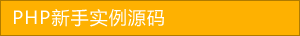﻿ php垃圾回收机制（gc）介绍 - php应用 - PHP粉丝网

# php垃圾回收机制（gc）介绍

##### 发布:smiling　来源: PHP粉丝网 　添加日期：2022-06-05 09:12:57　浏览: 评论：0

php的编译原理：

ze（zend engine）调用词法分析器把php 代码去空格，注释后分割成一个个token，ze调用语法分析器再对token处理形成opcode，opcode以op array形式存在，ze最后执行op array输出结果。

refcount：引用技术器，可以理解为指向该个容器的指针个数吧。

is_ref：是否被引用（只可能是0或者1）

1. <?php
2.
3. \$a = 'aa'
4.
5. xdebug_debug_zval(a);  //(refcount=1, is_ref=0),string 'aa' (length=6)
6.
7. \$b = \$a;
8.
9. //以下的两个其实是一个变量容器
10.
11. xdebug_debug_zval(a); //(refcount=2, is_ref=0),string 'aa' (length=6)
12.
13. xdebug_debug_zval(b); //(refcount=2, is_ref=0),string 'aa' (length=6)
14.
15. unset(\$b);  //对变量容器 refcount 减1xdebug_debug_zval(a); //(refcount=1, is_ref=0),string 'aa' (length=6)
16.
17. xdebug_debug_zval(b); //b: no such symbol  b变量被销毁，指向被断掉，如果对应容器的引用技术为零，那么该块儿内存被回收
18.
19. \$b = \$a
20.
21. \$b = 'bb'
22.
23. xdebug_debug_zval(a); //(refcount=1, is_ref=0),string 'aa' (length=6)
24.
25. xdebug_debug_zval(b); //(refcount=1, is_ref=0),string 'aa' (length=6)  重新申请一个变量容器存储b，a的变量容器引用减1

1. <?php
2.
3. \$a = 'aa'
4.
5. xdebug_debug_zval('a');  //(refcount=1, is_ref=0),string 'aa' (length=2)
6.
7. \$b = & \$a;//变量容器的引用技术加1，引用标记置为1xdebug_debug_zval('a');  //(refcount=2, is_ref=1),string 'aa' (length=2)
8.
9. xdebug_debug_zval('b');  //(refcount=2, is_ref=1),string 'aa' (length=2)
10.
11. \$b = '123';
12.
13. //php会发现，该容器变量是引用（is_ref），所以容器变量不用像赋值那样再申请一个
14.
15. xdebug_debug_zval('a');  //(refcount=2, is_ref=1),string '123' (length=2)
16.
17. xdebug_debug_zval('b');  //(refcount=2, is_ref=1),string '123' (length=2)
18.
19. unset(\$b);//变量容器应用计数减1，引用为零
20.
21. xdebug_debug_zval('a');  //(refcount=1, is_ref=0),string '123' (length=2)
22.
23. xdebug_debug_zval('b'); // b: no such symbol

1. <?php
2.
3. \$a = 'aa'
4.
5. \$b = &\$a
6.
7. \$c = &\$a;//可以看到引用refCount是3，is_ref永远是1xdebug_debug_zval('a'); //(refcount=3, is_ref=1),string 'aa' (length=2)
8.
9. xdebug_debug_zval('b'); //(refcount=3, is_ref=1),string 'aa' (length=2)
10.
11. xdebug_debug_zval('c'); //(refcount=3, is_ref=1),string 'aa' (length=2)
12.
13. unset(\$b);//我们期待的bug没有出现,只是refcount减1，is_ref还是1xdebug_debug_zval('a'); //(refcount=2, is_ref=1),string 'aa' (length=2)
14.
15. xdebug_debug_zval('b'); // b: no such symbol
16.
17. xdebug_debug_zval('c'); //(refcount=2, is_ref=1),string 'aa' (length=2)
18. //那php它怎么知道这个容器还有引用，毕竟is_ref仍然是1，不能计数，那么现在refcount就起作用了，是它告诉php，该变量有几个引用，但问题又来了，如果我干点坏事，在引用的时候，又赋值，它会不会有bug
19.
20. \$e = \$a;//我们看到期望的bug还是没出现，这时候再赋值，就不像直接赋值那么简单refcount加1了，而是申请了一个新的变量容器
21.
22. xdebug_debug_zval('a'); //(refcount=2, is_ref=1),string 'aa' (length=2)
23.
24. xdebug_debug_zval('e'); //(refcount=1, is_ref=0),string 'aa' (length=2)

unset和赋值null都能回收变量么？很多人都错认为，这两个都能回收变量空间，其实错了，null只是把变量占用的空间变小了，从回收上来说，该容器依然存在。

1. <?php
2.
3. \$a = 'aa'
4.
5. \$b = \$a
6.
7. \$b = null;
8.
9. //又申请了一个变量容器
10.
11. xdebug_debug_zval('a');  //(refcount=1, is_ref=0),string 'aa' (length=2)
12.
13. xdebug_debug_zval('b');  //(refcount=1, is_ref=0),null   变量空间并没被回收
14.
15. unset(\$b);
16.
17. //这时候才释放了b变量容器的空间
18.
19. xdebug_debug_zval('a');  //(refcount=1, is_ref=0),string 'aa' (length=2)
20.
21. xdebug_debug_zval('b');  //b: no such symbol

## 相关文章